### Chemical and Process Engineering Resources## Using Equivalent Lengths of Valves and Fittings

Nov 08 2010 01:20 PM | pleckner in Fluid Flow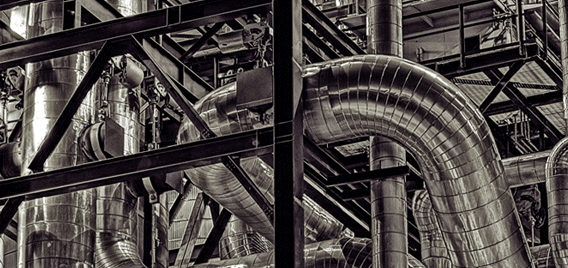One of the most basic calculations performed by any process engineer, whether in design or in the plant, is line sizing and pipeline pressure loss. Typically known are the flow rate, temperature and corresponding viscosity and specific gravity of the fluid that will flow through the pipe.

These properties are entered into a computer program or spreadsheet along with some pipe physical data (pipe schedule and roughness factor)

and out pops a series of line sizes with associated Reynolds Number, velocity, friction factor and pressure drop per linear dimension. The pipe size is then selected based on a compromise between the velocity and the pressure drop. With the line now sized and the pressure drop per linear dimension determined, the pressure loss from the inlet to the outlet of the pipe can be calculated.

Calculating Pressure Drop

The most commonly used equation for determining pressure drop in a straight pipe is the Darcy Weisbach equation. One common form of the equation which gives pressure drop in terms of feet of head is given below:Eq. (1)

The term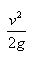is commonly referred to as the Velocity Head.

Another common form of the Darcy Weisbach equation that is most often used by engineers because it gives pressure drop in units of pounds per square inch (psi) is: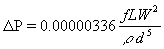Eq. (2)

To obtain pressure drop in units of psi/100 ft, the value of 100 replaces L in Equation 2.

The total pressure drop in the pipe is typically calculated using these five steps.

1. Determine the total length of all horizontal and vertical straight pipe runs.
2. Determine the number of valves and fittings in the pipe. For example, there may be two gate valves, a 90o elbow and a flow thru tee.
3. Determine the means of incorporating the valves and fittings into the Darcy equation. To accomplish this, most engineers use a table of equivalent lengths. This table lists the valve and fitting and an associated length of straight pipe of the same diameter, which will incur the same pressure loss as that valve or fitting. For example, if a 2" 90o elbow were to produce a pressure drop of 1 psi, the equivalent length would be a length of 2" straight pipe that would also give a pressure drop of 1 psi. The engineer then multiplies the quantity of each type of valve and fitting by its respective equivalent length and adds them together.
4. The total equivalent length is usually added to the total straight pipe length obtained in step one to give a total pipe equivalent length.
5. This total pipe equivalent length is then substituted for L in Equation 2 to obtain the pressure drop in the pipe.

See any problems with this method?

### Fluid Flow Articleslidachang

yeal,thank you very much,i got a lot from  this topic.ksb
In Crane Tech Paper 410 (1982 version), Example 4-14 shows a 5"x4" Reducing Elbow which they model as a straight size 5" elbow and a sudden enlargement 4" to 5". In step 4 of Example 4-14, they calculate a pressure increase (negative pressure drop) according to the Bernoulli theorem, because the water slows down as it flows from the smaller diameter to the larger diameter. I was wondering why you didn't include the velocity change in your Sulfuric Acid example since it flows from the 1" pump discharge nozzle through the reducer to the 3" pipe. Since the velocity in the 1" nozzle is 31.22 ft/s, the Bernoulli dP would be -11.72 psi plus the frictional dP of 7.24 psi for a total pressure drop of -4.48 psi which is a pressure increase.I'm a bit confused by the preferred equations to be used. The only equation that produces the 11.73 psi dP for the system described is Equation 2, yet Equations 6 & 7 seem to be preferred.

•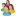Industrial Forum

•Student Forum

•Refining Forum

•Simulation Forum

•Relief Devices Forum

•Tank Venting Forum

•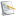Separation Technology

•Calculations and Tips

•Fluid Flow

•Heat Transfer

•Maintenance and Repair

•Utilities

•Safety and Pressure Relief

•Bulk Solids

•Process and Reactions

•Energy

•Other Topics

•For Students

•Physical Properties

•Archived Articles